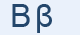Greek Letter Beta (Βήτα - Β)"Beta" is supposed to be the picture of a house, with two stories. "Beta" has the same ancestors with "Beth", Hebrew for "house", given to many Jewish congregations.

"B" Letter in mathematics and in science

Β represents:

the Beta function.

β represents:

• the second angle in a triangle.
• one root of a quadratic equation, where α represents the other
• the ratio of collector current to base current in a bipolar junction transistor (BJT) in electronics
• the false negative rate in statistics
• the beta coefficient, the non-diversifiable risk, of an asset in mathematical finance
• the sideslip angle of an airplane
• the first-order effects of variations in Coriolis force with latitude in planetary dynamics
• a beta particle (e-)
• sound intensity

Greek words with "β"

βοήθεια, βάλλω